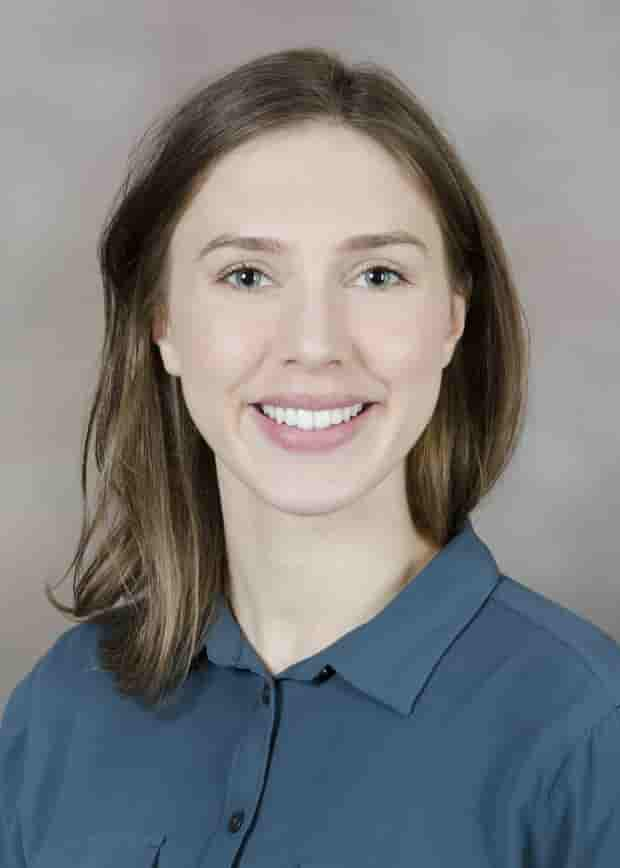×

# A Method for Assessing Confidence of Dual-Contrast Photon-Counting CT Material Quantification

## C Leary*, T Griglock, Oregon Health & Science Univ, Portland, OR

•C Leary

# Presentations

(Sunday, 7/12/2020)   [Eastern Time (GMT-4)]

Room: AAPM ePoster Library

Purpose: Multi-bin Photon-counting CT (PCCT) can quantify multiple materials at once, and accuracy of PCCT material quantification should be assessed. We present a method to propagate error through a linear material-decomposition system to assess confidence for dual-material quantification.

Methods: Four concentrations were scanned for iodine and gadolinium calibration. A sample mixture of 4.9mgI/ml and 5.3mgGd/ml was scanned with a Mars Small Animal Scanner (energy-thresholds: 20, 33, 50, 80, 120 keV) to test material quantification. ICP-MS was used to obtain accuracy of <0.5mg/ml.

A linear system, Ax=b, was used for post-reconstruction material-basis decomposition. For visualization of graphs on the iodine-gadolinium concentration plane, the attenuation contribution of water was subtracted to define “material-only attenuation”, which is a good approximation at small concentrations. The effect of subtraction on standard deviations was accounted for.

A, Calibration matrix: Each entry represents material-only attenuation per concentration for each material and energy-bin. A Monte Carlo method was used to fit 500 lines to the measured attenuation and SD of the calibration series. The calibration matrix contains the mean and SD of the slopes.
b, Measurement vector: Contains the mean and SD of the material-only attenuation in each energy bin of the sample scan.
x, Calculated concentration vector: 1000 solutions to the linear system were generated via Monte Carlo method. Solutions were plotted on the iodine-gadolinium concentration plane.
Confidence intervals: A multivariate gaussian was fit to the simulated concentration solutions, and 65% and 95% confidence intervals were plotted.

Results: Confidence intervals were visualized on the iodine-gadolinium concentration plane. The distance between known and calculated sample concentration was small, but the confidence intervals were large. This shows that pixel variation in concentration measurement may be large.

Conclusion: Empirical data and Monte-Carlo method can be used to propagate error through a linear system to assess confidence of PCCT dual-material quantification.

Keywords

Taxonomy

IM- CT: Quantitative imaging/analysis

Contact Email